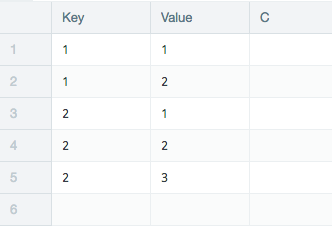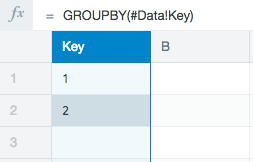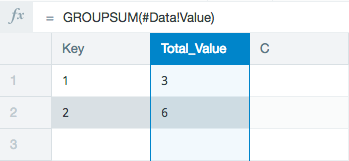## How to Replicate the SUMIF Function in Datameer

Goal

To use the popular SUMIF function which sums values within a range that also meets a specified criteria.

Learn

SUMIF can be accomplished in Datameer by combining the two separate functions GROUPSUM and IF

Example

You are working with the data below.The first step is to create groups that contain the values you want to sum.

This example has two groups, "Key 1" and "Key 2".If you were to do a GROUPSUM for these groups, "Key 1" has the sum of 3 and "Key 2" has sum 6.But, if you want to only sum the values within the groups that meet a specific criteria, the IF statement needs to be added to the formula.

This example wants to exclude any values less than 2 from the total sum.

The formula looks like this:

 GROUPSUM( IF( (#Data!Value >= 2); #Data!Value; 0) )

This formula now checks the values from the Value column on the Data sheet to see IF they are greater than or equal to 2. If not, the value is changed to 0.

The results of this formula executes the GROUPSUM function with the specified conditional statement.

Both the groups had a value that was 1. This value was changed to 0 as specified in the IF statement. The new sum of the groups in this example are displayed in the Total_Value1 column.